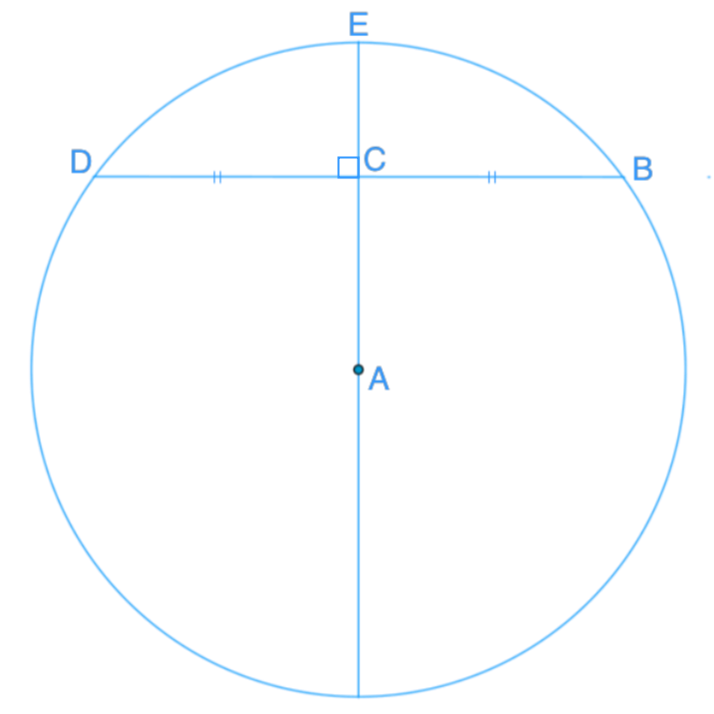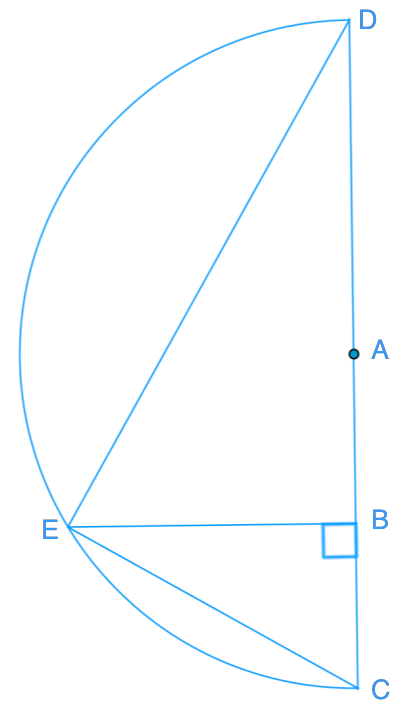# Chord properties

### Chord properties

In a circle, when a line passes through the center of the circle and is perpendicular to a chord, the line will bisect the chord. In other words, the perpendicular bisector of a chord always passes through the center of the circle.

#### Lessons

• Introduction
a)
1. What is a chord?
2. What is a tangent?

b)
What are the properties of a chord?

• 1.
If AC is 4 cm and BD is 15 cm, what is the diameter of the circle?• 2.
Zoe wants to set up her round dining table by putting a candle in the centre of it. How can she do that by using a tangent? Explain your answer with a diagram.

• 3.
Below is a segment of a circle, and A is the center of the circle. The radius is 15 cm, BC is 7.5 cm, and DE is 24 cm.Determine.
a)
$\angle$CED

b)
CE

c)
BE